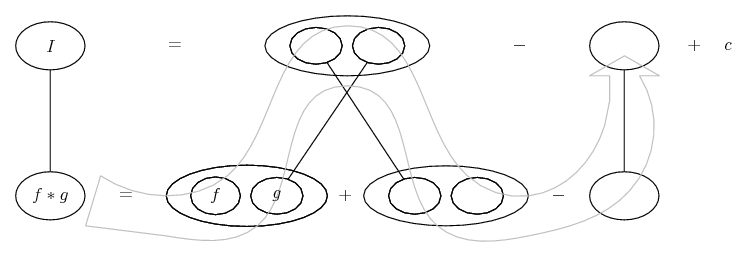# Thread: integration by parts issue

1. ## integration by parts issue

if you click the link: integrate x&#94;2e&#94;&#40;4x&#41; - Wolfram|Alpha

youll see the problem im working on. I dont understand how W.A got +1/8 before the line that says "the integral of e^4x is..."

can someone explain it to me? ive tried doing this multiple ways, but it doesnt make sense, and the answer is correct

2. ## Re: integration by parts issueOriginally Posted by NecroWinterif you click the link: integrate x^2e^(4x) - Wolfram|Alpha

youll see the problem im working on. I dont understand how W.A got +1/8 before the line that says "the integral of e^4x is..."

can someone explain it to me? ive tried doing this multiple ways, but it doesnt make sense, and the answer is correct
$\displaystyle \frac{1}{2} \cdot I=\frac{1}{2} \cdot \int e^{4x} \cdot x \, dx$

$\displaystyle x=u \Rightarrow dx=du$

$\displaystyle e^{4x} dx=dv \Rightarrow 4x=t \Rightarrow 4 dx=dt \Rightarrow dx = \frac{1}{4} dt \Rightarrow$

$\displaystyle \Rightarrow \frac{1}{4} e^t dt =dv \Rightarrow \frac{1}{4} \cdot \int e^t \,dt = \int dv \Rightarrow v= \frac{1}{4} \cdot e^t \Rightarrow v = \frac{1}{4} \cdot e^{4x}$

$\displaystyle \frac{1}{2} \cdot I= \frac{1}{8} \cdot x \cdot e^{4x}-\frac{1}{8} \cdot \int e^{4x} \,dx$

3. ## Re: integration by parts issue

Just in case a picture helps...... where (key in spoiler) ...

Spoiler:... is the chain rule. Straight continuous lines differentiate downwards (integrate up) with respect to the main variable (in this case x), and the straight dashed line similarly but with respect to the dashed balloon expression (the inner function of the composite which is subject to the chain rule).

But this is wrapped inside the legs-uncrossed version of...... the product rule, where, again, straight continuous lines are differentiating downwards with respect to x.... is lazy integration by parts, doing without u and v.

Larger

__________________________________________________ __________

Don't integrate - balloontegrate!

Balloon Calculus; standard integrals, derivatives and methods

Balloon Calculus Drawing with LaTeX and Asymptote!

#### Search Tags

integration, issue, parts